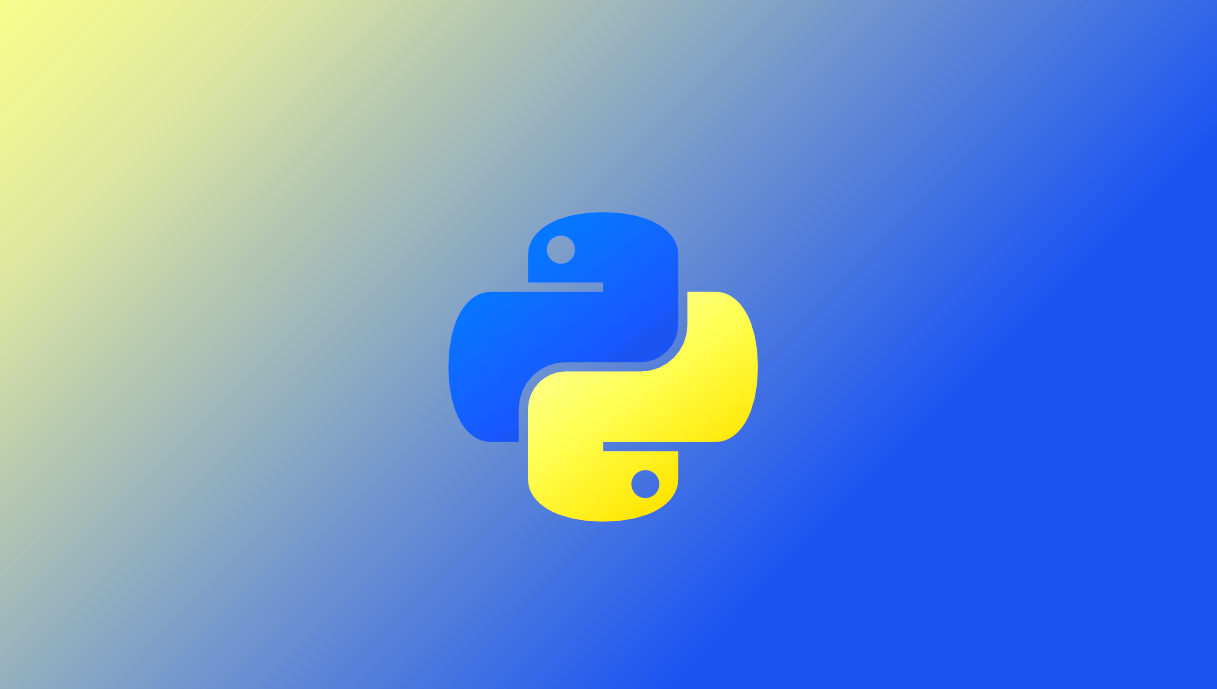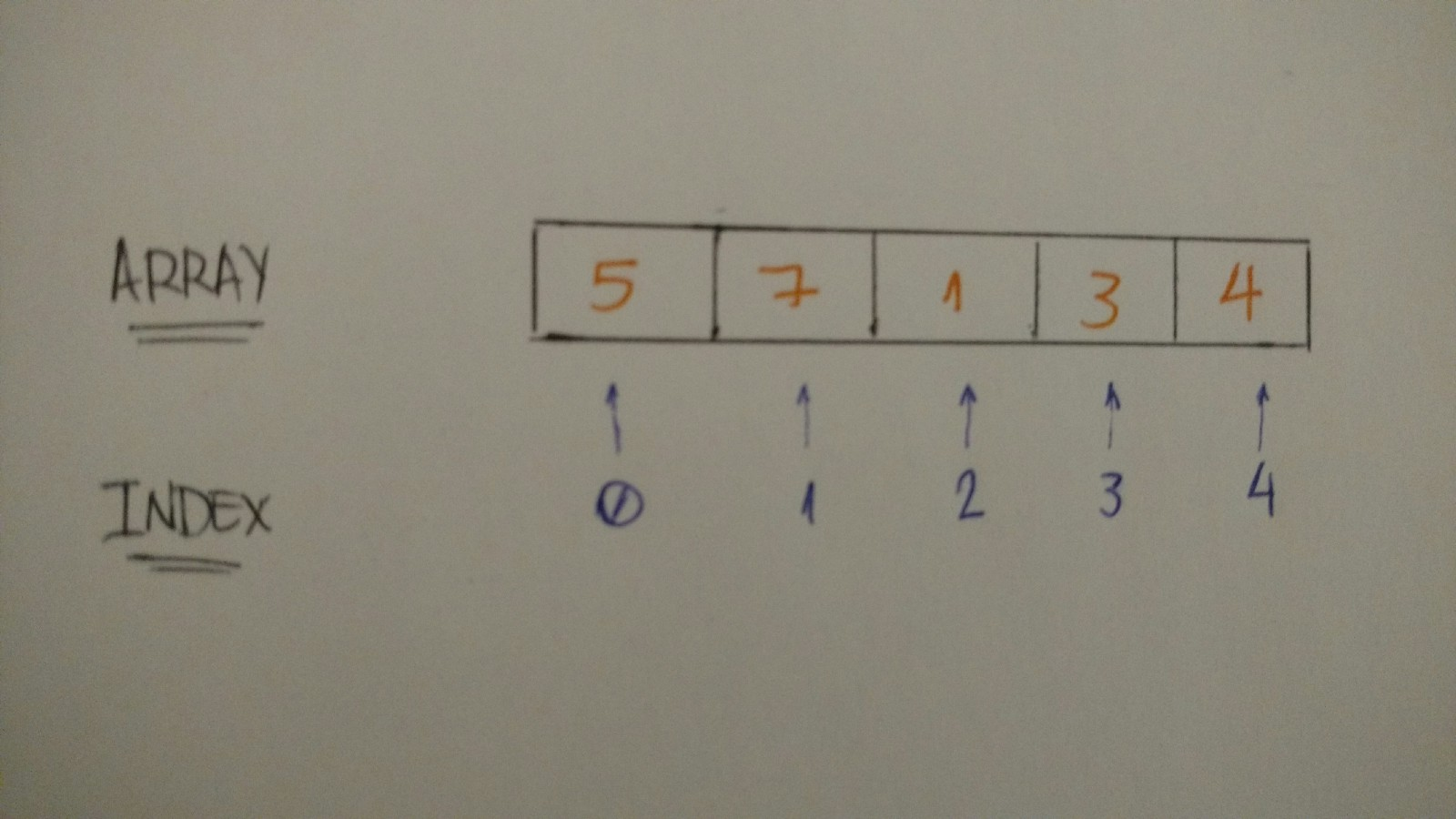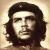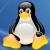从 Zero 到 Hero ，一文掌握 Python已翻译 100%

oschina 投递于 2017/11/22 11:27 (共 17 段, 翻译完成于 11-27)

参与翻译 (5人) : rever4433, Tocy, 承蒙, 南宫冰郁, 透过树叶的光Python 基础

1.变量

Python 中定义一个变量并为它赋值是很容易的。假如你想存储数字 1 到变量 “one” ，让我们试试看：

one = 1

two = 2
some_number = 10000

# booleans
true_boolean = True
false_boolean = False

# string
my_name = "Leandro Tk"

# float
book_price = 15.80

2.控制流程：条件语句

If” 使用一个表达式来判断一个语句是 True 还是 False ，如果是 True ，那么执行 if 内的代码，例子如下：

if True:
print("Hello Python If")

if 2 > 1:
print("2 is greater than 1")

if 1 > 2:
print("1 is greater than 2")
else:
print("1 is not greater than 2")

if 1 > 2:
print("1 is greater than 2")
elif 2 > 1:
print("1 is not greater than 2")
else:
print("1 is equal to 2")

3. 循环和迭代

While 循环：当语句是 True 时，while 内部的代码块会执行。所以下面这段代码会打印出 到 10 。

num = 1

while num <= 10:
print(num)
num += 1

while 循环需要循环条件，如果条件一直是 True ，它将会一直迭代，当 num 的值为 11 时，循环条件为 false 。

loop_condition = True

while loop_condition:
print("Loop Condition keeps: %s" %(loop_condition))
loop_condition = False

For 循环：你可以在代码块上应用变量 “num” ，而 “for” 语句将为你迭代它。此代码将打印与 while 中相同的代码：从 1 到 10 。

for i in range(1, 11):
print(i)

List：集合 | 数组 | 数据结构

my_integers = [1, 2, 3, 4, 5]my_integers = [5, 7, 1, 3, 4]
print(my_integers) # 5
print(my_integers) # 7
print(my_integers) # 4

relatives_names = [
"Toshiaki",
"Juliana",
"Yuji",
"Bruno",
"Kaio"
]

print(relatives_names) # Kaio

bookshelf = []
bookshelf.append("The Effective Engineer")
bookshelf.append("The 4 Hour Work Week")
print(bookshelf) # The Effective Engineer
print(bookshelf) # The 4 Hour Work Week

字典：Key-Value 数据结构

dictionary_example = {
"key1": "value1",
"key2": "value2",
"key3": "value3"
}

Key 是指向 value 的索引。我们如何访问字典中的 value 呢？你应该猜到了，那就是使用 key 。我们试一下：

dictionary_tk = {
"name": "Leandro",
"nickname": "Tk",
"nationality": "Brazilian"
}

print("My name is %s" %(dictionary_tk["name"])) # My name is Leandro
print("But you can call me %s" %(dictionary_tk["nickname"])) # But you can call me Tk
print("And by the way I'm %s" %(dictionary_tk["nationality"])) # And by the way I'm Brazilian

dictionary_tk = {
"name": "Leandro",
"nickname": "Tk",
"nationality": "Brazilian",
"age": 24
}

print("My name is %s" %(dictionary_tk["name"])) # My name is Leandro
print("But you can call me %s" %(dictionary_tk["nickname"])) # But you can call me Tk
print("And by the way I'm %i and %s" %(dictionary_tk["age"], dictionary_tk["nationality"])) # And by the way I'm Brazilian

迭代：通过数据结构进行循环

bookshelf = [
"The Effective Engineer",
"The 4 hours work week",
"Zero to One",
"Lean Startup",
"Hooked"
]

for book in bookshelf:
print(book)

dictionary = { "some_key": "some_value" }

for key in dictionary:
print("%s --> %s" %(key, dictionary[key]))

# some_key --> some_value

dictionary = { "some_key": "some_value" }

for key, value in dictionary.items():
print("%s --> %s" %(key, value))

# some_key --> some_value

dictionary_tk = {
"name": "Leandro",
"nickname": "Tk",
"nationality": "Brazilian",
"age": 24
}

for attribute, value in dictionary_tk.items():
print("My %s is %s" %(attribute, value))

# My name is Leandro
# My nickname is Tk
# My nationality is Brazilian
# My age is 24

类&对象

一些理论:

Python 面向对象编程模式：ON

Python，作为一种面向对象编程语言，存在这样的概念：对象

class Vehicle:
pass

car = Vehicle()
print(car) # <__main__.Vehicle instance at 0x7fb1de6c2638>

class Vehicle:
def __init__(self, number_of_wheels, type_of_tank, seating_capacity, maximum_velocity):
self.number_of_wheels = number_of_wheels
self.type_of_tank = type_of_tank
self.seating_capacity = seating_capacity
self.maximum_velocity = maximum_velocity

tesla_model_s = Vehicle(4, 'electric', 5, 250)

评论(14)

l引用来自“吕懂不”的评论

if 1 > 2:
print("1 is greater than 2")elif 2 > 1:
print("1 is not greater than 2")else:
print("1 is equal to 2")

引用来自“听风的小猪”的评论